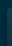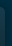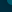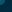# Stovall James - Good Day Chords & Tabs## Good Day Chords & Tabs

Version: 1 Type: Tab
`Good Day Tab`
```james stovall
good day

actually its a mandolin tab its the first ive done so here goes

intro
=2=2=2=2=2=2=235320====
=0=0=3=3=0=0=======232=
=0=0=0=0=0=0===========
=2=2=0=0=2=2===========
[ Tab from: https://www.guitartabs.cc/tabs/s/stovall_james/good_day_tab.html ]
verse/chours
=2==55==7==2357532==========
=2==55==7===================
=2==55==7===================
=2==55==7===================

breakdown
=xx==12*==
=xx==12*==
=xx==12*==repeat 4 times
=xx==12*==

*/harmonics

this is my song i fooled around with at an fm 1863 concert
go to their website at fm1863.com
```